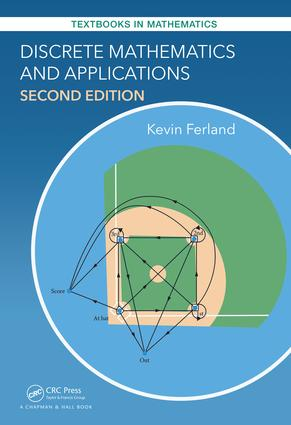# Discrete Mathematics and Applications

## 2nd Edition

Chapman and Hall/CRC

916 pages | 893 B/W Illus.

##### Purchasing Options:\$ = USD
Hardback: 9781498730655
pub: 2017-05-01
\$105.00
x

FREE Standard Shipping!

### Description

This book is intended for a one-semester course in discrete mathematics. Such a course is typically taken by mathematics, mathematics education, and computer science majors, usually in their sophomore year. Calculus is not a prerequisite to use this book. Part one focuses on how to write proofs, then moves on to topics in number theory, employing set theory in the process. Part two focuses on computations, combinatorics, graph theory, trees, and algorithms.

I Proofs

Logic and Sets

Statement Forms and Logical Equivalences

Set Notation

Quantifiers

Set Operations and Identities

Valid Arguments

Basic Proof Writing

Direct Demonstration

General Demonstration (Part 1)

General Demonstration (Part 2)

Indirect Arguments

Splitting into Cases

Elementary Number Theory

Divisors

Well-Ordering, Division, and Codes

Euclid's Algorithm and Lemma

Rational and Irrational Numbers

Modular Arithmetic and Encryption

Indexed by Integers

Sequences, Indexing, and Recursion

Sigma Notation

Mathematical Induction, An Introduction

Induction and Summations

Strong Induction

The Binomial Theorem

Relations

General Relations

Special Relations on Sets

Basics of Functions

Special Functions

General Set Constructions

Cardinality

II Combinatorics

Basic Counting

The Multiplication Principle

Permutations and Combinations

Probability

Applications of Combinations

Correcting for Overcounting

More Counting

Inclusion-Exclusion

Multinomial Coe□cients

Generating Functions

Counting Orbits

Combinatorial Arguments

Basic Graph Theory

Motivation and Introduction

Special Graphs

Matrices

Isomorphisms

Invariants

Directed Graphs and Markov Chains

Graph Properties

Connectivity

Euler Circuits

Hamiltonian Cycles

Planar Graphs

Chromatic Number

Trees and Algorithms

Trees

Search Trees

Weighted Trees

Analysis of Algorithms (Part 1)

Analysis of Algorithms (Part 2)

A Assumed Properties of Z and R

B Pseudocode

C Answers to Selected Exercises

### About the Originator

#### Kevin Ferland

Bloomsburg, PA, USA